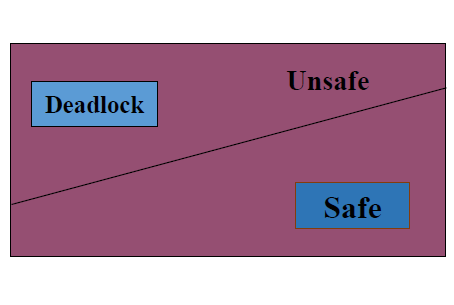# Deadlock Avoidance and Bankers Algorithm in OS

It is one of the methods of dynamically escaping from the deadlocks. The word dynamically means ‘Online’. In this scheme, if a process request for resources the avoidance algorithm checks before the allocation of resources about the state of the system. If the state is safe the system allocates the resources to the requesting process otherwise (unsafe) do not allocate the resources. So taking care before the allocation is said to be deadlock avoidance.

Safe state: No deadlock will happen, even we allocate the resources to requesting processes.Deadlock avoidance/deadlock avoidance in os

Banker’s algorithm /bankers algorithm

• It is a deadlock avoidance algorithm, the name was chosen because the bank never allocates more than the available cash.
• Multiple instances.
• Each process must a priori claim maximum use.
• When a process requests a resource it may have to wait.
• When a process gets all its resources it must return them in a finite amount of time.

Data Structures for the Banker’s AlgorithmData Structures for the Banker’s AlgorithmSafety Algorithm for Banker’s algorithm

Example of Banker’s algorithm /banker's algorithm in osbanker's algorithm in os

Now, we have to calculate the available matrix of the resources.
Available = Total-Allocation
R1 = 10 - (2+3+2)=3
R2 = 5- (1+1) = 3
R3 = 7 – (2+1+2) = 2
Available matrix is (3,3,2)

Now the snapshot is modified:banker's algorithm in os available matrix

Now we have to calculate the need matrix:banker's algorithm in os need matrix

• Now we know the need matrix and available matrix, so we can apply the banker’s algorithm on this example. Search the need matrix from P0 to P4 which is less than the available matrix, (1,2,2)<(3,3,2). Then allocate the requesting resource to P1. Now the process P1 may allocate (2,0,0+1,2,2)=(3,2,2).

• After the completion of job P1, it releases the total resources, then available resource is (3,3,2+2,0,0)=(5,3,2).

• Search the need matrix again, which is less than the available (0,1,1)<(5,3,2). To allocate resources to P3. after the completion of P3, it releases all its resources. Then the available =(available+allocation). It is (5,3,2+2,1,1)=7,4,3. continues this process until we find the safe state otherwise wait. The safe state sequence for this problem is <P1, P3, P4, P2, P0>.